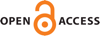#### Vol. 10, No. 4, 2017

 Download this articleFor screen For printingRecent IssuesThe Journal About the Journal Editorial Board Editors’ Interests Subscriptions Submission Guidelines Submission Form Policies for Authors Ethics Statement ISSN: 1944-4184 (e-only) ISSN: 1944-4176 (print) Author Index Coming Soon Other MSP Journals
The $H$-linked degree-sum parameter for special graph families

### Lydia East Kenney and Jeffrey Scott Powell

Vol. 10 (2017), No. 4, 707–720##### Abstract

For a fixed graph $H$, a graph $G$ is $H$-linked if any injection $f:V\left(H\right)\to V\left(G\right)$ can be extended to an $H$-subdivision in $G$. The concept of $H$-linked generalizes several well-known graph theory concepts such as $k$-connected, $k$-linked, and $k$-ordered. In 2012, Ferrara et al. proved a sharp ${\sigma }_{2}$ (or degree-sum) bound for a graph to be $H$-linked. In particular, they proved that any graph $G$ with $n>20|E\left(H\right)|$ vertices and ${\sigma }_{2}\left(G\right)\ge n+a\left(H\right)-2$ is $H$-linked, where $a\left(H\right)$ is a parameter maximized over certain partitions of $V\left(H\right)$. However, they do not discuss the calculation of $a\left(H\right)$ in their work. In this paper, we prove the exact value of $a\left(H\right)$ in the cases when $H$ is a path, a cycle, a union of stars, a complete graph, and a complete bipartite graph. Several of these results lead to new degree-sum conditions for particular graph classes while others provide alternate proofs of previously known degree-sum conditions.

##### Keywords
H-linked, path, cycle, degree-sum, Ore condition
##### Mathematical Subject Classification 2010
Primary: 05C35, 05C38
Secondary: 05C83
##### Milestones